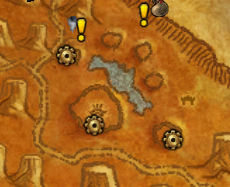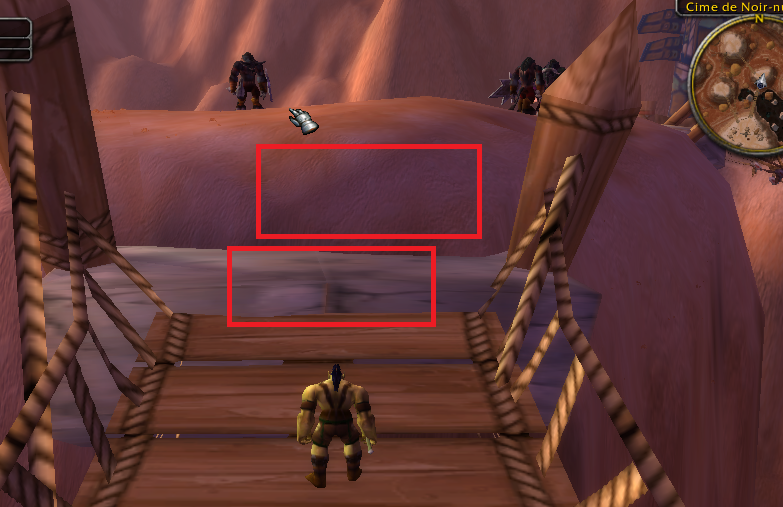• ```[N] 18:25:22 - [Path-Finding] FindPath from -5163,156 ; -1854,908 ; -52,30281 ; "None" to -5139,71 ; -1918,91 ; 89,1875 ; "None" (Kalimdor)
[N] 18:25:24 - [Server] [RD]  findPath out of nodes
[N] 18:25:24 - [Server] [Path-Finding] Partial result: -5163,156 ; -1854,908 ; -52,30281 ; "None" (Kalimdor_35,47795_41,68092) to -5139,71 ; -1918,91 ; 89,1875 ; "None" (Kalimdor_35,59796_41,63696)
[N] 18:25:24 - [Server] [Path-Finding] Partial result: -5139,71 ; -1918,91 ; 89,1875 ; "None" (Kalimdor_35,59796_41,63696) to -5159,037 ; -1866,435 ; -47,92997 ; "None" (Kalimdor_35,49957_41,67319)
[N] 18:25:24 - [Path-Finding] Path Count: 17 (265,0005y)
[N] 18:25:31 - [MovementManager] Think we are stuck
[N] 18:25:31 - [MovementManager] Trying something funny, hang on
[D] 18:25:31 - [MovementManager] Avoid wall: StrafeLeft
[N] 18:25:32 - [MovementManager] Think we are stuck
[N] 18:25:32 - [MovementManager] Trying something funny, hang on
[D] 18:25:32 - [MovementManager] Avoid wall: StrafeLeft
[N] 18:25:34 - [MovementManager] Report navmesh poly to avoid it```

so.. bot tries to walk up a long cliff, as if ignoring Z coordinate completly.

- i think there are more places like this in 1k needles, is there some sort of bug that just recently started?

## User Feedback

adding more details - bot seems to be unable to path to any of these three gears in this picture (quest is grimtotem spying)

specific coordinates ( try to navigate these from anywhere outside of these three big rocks/mountains, the problem will occur)

<Vector3 X="-4922.240" Y="-1831.890" Z="80.820"/>
<Vector3 X="-5139.710" Y="-1918.910" Z="89.188"/>
<Vector3 X="-5184.790" Y="-2164.450" Z="94.634"/>##### Share on other sites

Hello, you need to use this offmeshesconnections ( "WRobot\Data\OffMeshConnections.xml" )

```  <OffMeshConnection>
<Path>
<Vector3 X="-4890.577" Y="-1975.606" Z="92.24278" />
<Vector3 X="-5021.466" Y="-1938.876" Z="90.04434" />
</Path>
<ContinentId>1</ContinentId>
<Name>Cime de Noir-nuage</Name>
</OffMeshConnection>
<OffMeshConnection>
<Path>
<Vector3 X="-5021.466" Y="-1938.876" Z="90.04434" />
<Vector3 X="-4891.924" Y="-1970.782" Z="91.50689" />
</Path>
<ContinentId>1</ContinentId>
<Name>Cime de Noir-nuage</Name>
</OffMeshConnection>
<OffMeshConnection>
<Path>
<Vector3 X="-5027.926" Y="-1931.706" Z="90.34178" />
<Vector3 X="-5004.158" Y="-1940.912" Z="84.85013" />
</Path>
<ContinentId>1</ContinentId>
<Name>Cime de Noir-nuage</Name>
</OffMeshConnection>
<OffMeshConnection>
<Path>
<Vector3 X="-5004.158" Y="-1940.912" Z="84.85013" />
<Vector3 X="-5023.804" Y="-1938.739" Z="90.57607" />
</Path>
<ContinentId>1</ContinentId>
<Name>Cime de Noir-nuage</Name>
</OffMeshConnection>
<OffMeshConnection>
<Path>
<Vector3 X="-4906.365" Y="-1969.007" Z="85.78034" />
<Vector3 X="-4891.267" Y="-1973.176" Z="91.93782" />
</Path>
<ContinentId>1</ContinentId>
<Name>Cime de Noir-nuage</Name>
</OffMeshConnection>
<OffMeshConnection>
<Path>
<Vector3 X="-4891.267" Y="-1973.176" Z="91.93782" />
<Vector3 X="-4906.328" Y="-1968.438" Z="85.74543" />
</Path>
<ContinentId>1</ContinentId>
<Name>Cime de Noir-nuage</Name>
</OffMeshConnection>
<OffMeshConnection>
<Path>
<Vector3 X="-5154.633" Y="-2129.755" Z="91.84631" />
<Vector3 X="-5149.066" Y="-2109.99" Z="88.07574" />
</Path>
<ContinentId>1</ContinentId>
<Name>Cime de Noir-nuage</Name>
</OffMeshConnection>
<OffMeshConnection>
<Path>
<Vector3 X="-5149.066" Y="-2109.99" Z="88.07574" />
<Vector3 X="-5154.358" Y="-2130.45" Z="91.88598" />
</Path>
<ContinentId>1</ContinentId>
<Name>Cime de Noir-nuage</Name>
</OffMeshConnection>
<OffMeshConnection>
<Path>
<Vector3 X="-5153.188" Y="-2128.675" Z="91.61093" />
<Vector3 X="-5119.792" Y="-1986.772" Z="87.88402" />
<Vector3 X="-5079.95" Y="-1946.185" Z="89.30199" />
<Vector3 X="-5030.777" Y="-1935.503" Z="90.53847" />
<Vector3 X="-4890.049" Y="-1971.698" Z="91.81448" />
</Path>
<ContinentId>1</ContinentId>
<Name>Cime de Noir-nuage</Name>
</OffMeshConnection>
<OffMeshConnection>
<Path>
<Vector3 X="-4890.049" Y="-1971.698" Z="91.81448" />
<Vector3 X="-5027.663" Y="-1937.253" Z="90.62695" />
<Vector3 X="-5088.373" Y="-1945.523" Z="88.93313" />
<Vector3 X="-5118.782" Y="-1992.137" Z="87.27573" />
<Vector3 X="-5153.819" Y="-2131.953" Z="91.95806" />
</Path>
<ContinentId>1</ContinentId>
<Name>Cime de Noir-nuage</Name>
</OffMeshConnection>```

Pathfinder stuck here: